# Multiple recursion

A form of recursion using several variables simultaneously. The set of values of these variables is ordered lexicographically. This definition subsumes numerous concrete recursive descriptions. If in such a description the unknown function is not substituted into itself, then it results in a primitive recursion. In general, multiple recursion leads outside the limits of the set of primitive recursive functions, since by a double recursion (performed with respect to two variables) it is possible to construct a function which is universal for the primitive recursive functions (similarly, for-recursive functions there is a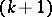-multiple universal function); cf. Universal function. All possible forms of multiple recursion can be reduced to the following normal form: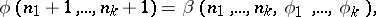where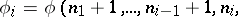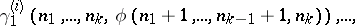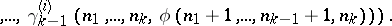How to Cite This Entry:
Multiple recursion. N.V. Belyakin (originator), Encyclopedia of Mathematics. URL: http://encyclopediaofmath.org/index.php?title=Multiple_recursion&oldid=15829
This text originally appeared in Encyclopedia of Mathematics - ISBN 1402006098